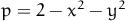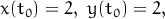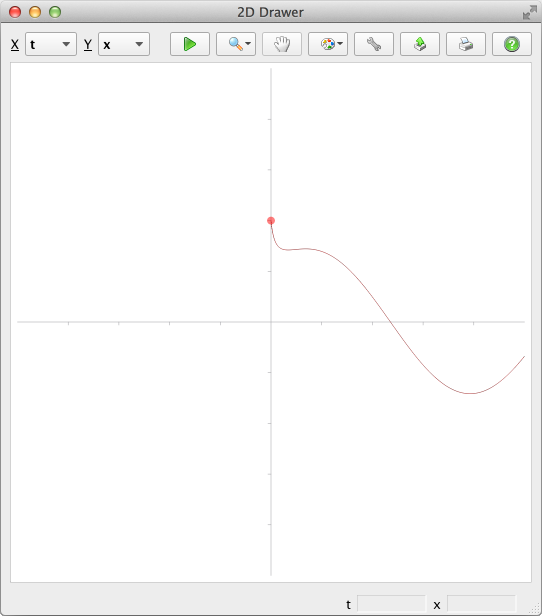### 4 Programming and Solving

Before solving your system of ordinary differential equations you need to program it in Runge. This section describes the way you can do that.

#### 4.1 System

1.2.
Choose dimension:3.
Choose independent variable. It should be valid identifier (i.e. a string beginning with letter):4.
Enter the system you’d like to solve. First column is for dependent variables (valid identifiers, i.e. strings beginning with letter), second one is for equations. Equations should be valid expressions containing arithmetic operators and elementary functions. You can also use macros (like macro “p” shown below) for repeating expressions:The following system is entered at this picture:#### 4.2 Parameters

1.
Choose solver. Not every solver works for a given system type:2.
Set start parameters, values and macros:Here you can set:

• Start t That’s the initial value of the independent variable.
• End t That’s the end value of the independent variable — last solution will be computed at this point.
• H Initial step. Real step will be computed according to precision required.
• Hmin Minimum step allowed. If precision can’t be reached even for this step, solver fails.
• Hmax Maximum step allowed.
• Eps Absolute precision required on each step.
• P Relative precision measure. If some component’s absolute value becomes greater than P, it gets normalized when precision compared to Eps.
• Macros List with macros. Here we havetherefore the final system turns to be• Dep. var. - Start Value Initial values for each dependent variable. In this particular case we have:where, see Start t above.

#### 4.3 Solution

1.
Click Start buttonto start solving the system. For big systems or long time ranges you might see progress barHere you can stop, pause and resume solving process by using appropriate buttons.

2.
Explore solutionEvery solution has an id starting from zero.

3.
Explore solution graphically (here you can select different variables for X and Y axes):#### 4.4 Export

Use Export feature to export solution to other programs like MS Excel or MATLABExcel should read it like this:MATLAB should read it like this:And after issuing a command like

>> plot(solution0(:,2), solution0(:,3));

it should render a plot: